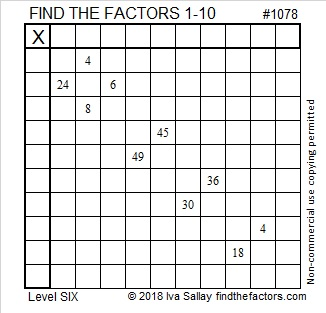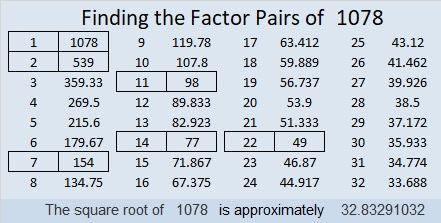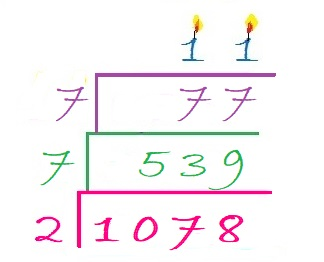# 1078 and Level 6

This Level 6 puzzle might be just a little bit easier than usual, so if you’ve never done this level before, be sure to try this one! You can succeed if you stick with it!Print the puzzles or type the solution in this excel file: 10-factors-1073-1079

Here are a few facts about the number 1078:

• 1078 is a composite number.
• Prime factorization: 1078 = 2 × 7 × 7 × 11, which can be written 1078 = 2 × 7² × 11
• The exponents in the prime factorization are 1, 2, and 1. Adding one to each and multiplying we get (1 + 1)(2 + 1)(1 + 1) = 2 × 3 × 2 = 12. Therefore 1078 has exactly 12 factors.
• Factors of 1078: 1, 2, 7, 11, 14, 22, 49, 77, 98, 154, 539, 1078
• Factor pairs: 1078 = 1 × 1078, 2 × 539, 7 × 154, 11 × 98, 14 × 77, or 22 × 49,
• Taking the factor pair with the largest square number factor, we get √1078 = (√49)(√22) = 7√22 ≈ 32.832911 – 0 + 7 – 8 = 0 so 1078 is divisible by 11.

Since 11 is its largest prime factor we can make a lovely factor cake for 1078:1078 is palindrome 4554 in BASE 6 because 4(6³) + 5(6²) + 5(6) + 4(1) = 1078

This site uses Akismet to reduce spam. Learn how your comment data is processed.